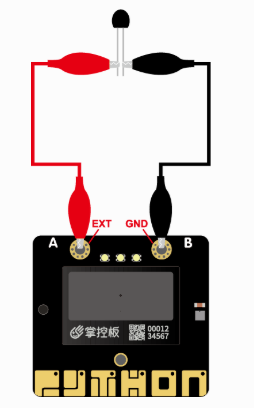# 数字IO

### 注意：

``除P2(只限数字输入)P3,P4,P10以外,其他引脚均可是使用数字输入、输出模式。有关更详细说明,请查看 :ref:`掌控板接口引脚说明<mPythonPindesc>` 。``

## 数字输入

``````    from mpython import *           # 导入mpython模块

p5=MPythonPin(5,PinMode.IN)     # 实例化MPythonPin,将按键a引脚(P5)设置为"PinMode.IN"模式

while True:
oled.DispChar("Button_a:%d" %value,30,20)   # 将读取到值显示至oled上
oled.show()                                  # 刷新
oled.fill(0)                                 # 清屏``````

### 注意：

``这时,你可以按下button a按键看下“按下”和“未按下”的读值。由于按键a电路做了上拉,所以“未按下”时输出为高电平, “按下”时输出为低电平。  ``
``````    from mpython import *
p5=MPythonPin(5,PinMode.IN) ``````

``    p5.read_digital()``

## 数字输出

``````    from mpython import *           # 导入mpython模块

p0=MPythonPin(0,PinMode.OUT)     # 实例化MPythonPin,将P0设置为"PinMode.OUT"模式

while True:
p0.write_digital(1)          # P0写高电平
sleep(1)                     #  延时
p0.write_digital(0)          # P0写高电平
sleep(1)                     #  延时``````

### 注意：

``上面需要使用到一块面包板、1个LED灯、mPython拓展板、杜邦线。LED灯的正极连接至掌控板的P0引脚,LED负极连接至掌控板的GND。  ``
``    p0=MPythonPin(0,PinMode.OUT)  ``

### 注解：

````MPythonPin`` 实例化。``mode`` 设置为 ``PinMode.OUT`` 数字输出模式。   ``

``````    p0.write_digital(1)
p0.write_digital(0)``````

### 注解：

``使用 ``write_digital(value)`` 方法对引脚写高低电平。其中 ``value`` 是电平值,“1”代表高电平,“0”代表低电平。   ``

## 外部中断

### 什么是中断呢？

``````在程序运行过程中，系统出现了一个必须由CPU立即处理的情况，此时，CPU暂时中止程序的执行转而处理这个新的情况的过程就叫做中断。

``````    from mpython import *           # 导入mpython模块
import music                    # 导入music模块
p5=MPythonPin(5,PinMode.IN)     # 实例化MPythonPin,将P5设置为"PinMode.IN"模式

def BuzzOn(_):                    # 定义中断的回调函数
music.play(music.BA_DING,wait=False)

p5.irq(trigger=Pin.IRQ_FALLING,handler=BuzzOn)            # 设置P5引脚中断的回调函数``````

### 提示：

``效果和时用 ``button_a.irq()`` 按键中断时一样的,button_a的中断也是使用到 ``Pin.irq`` 的方法。  ``

``    p5=MPythonPin(5,PinMode.IN) ``

``````    def BuzzOn(_):
music.play(music.BA_DING,wait=False)``````

### 注解：

``    p5.irq(trigger=Pin.IRQ_FALLING,handler=BuzzOn)``

### 注解：

``````我们将P5设置为仅在下降沿触发  ``Pin.IRQ_FALLING`` （当它从高电平变为低电平时）。设置回调函数
handler="你定义中断处理的回调函数"。更详细的触发方式，请查阅 :ref:`MPythonPin.irq <MPythonPin.irq>` 。   ``````

# 模拟IO

### 注意：

``你可以查阅 :ref:`掌控板接口引脚说明<mPythonPindesc>` ,了解可供使用的模拟引脚 。``

## 模拟输入

### 什么是模拟输入呢？

``模拟输入是将模拟信号转换为数字信号，简称ADC。   ``

``````    from mpython import *           # 导入mpython模块

p0=MPythonPin(0,PinMode.ANALOG)     # 实例化MPythonPin,将P0设置为"PinMode.ANALOG"模式
while True:
oled.DispChar("analog:%d" %value,30,20)
oled.show()
oled.fill(0)``````
``````    from mpython import *
p0=MPythonPin(0,PinMode.ANALOG)``````

### 注解:

````MPythonPin`` 实例化。``mode`` 设置为 ``PinMode.ANALOG`` 模拟输入模式。``

``    p0.read_analog()``

### 注解:

``因为adc采样数据宽度为12bit，所以满量程为4095。``

## EXT鳄鱼夹

EXT连接是掌控板的P3引脚:

``````    from mpython import *           # 导入mpython模块

p3=MPythonPin(3,PinMode.ANALOG)     # 实例化MPythonPin,将P3设置为"PinMode.ANALOG"模式
while True:
oled.DispChar("analog:%d" %value,30,20)
oled.show()
oled.fill(0)``````## 模拟输出模拟输出

### 什么是模拟输出呢？

``````电路板的引脚不能像音频放大器那样输出模拟信号 - 通过调制引脚上的电压。这些引脚只能使能全3.3V输出，或者将其下拉至0V。

``````    from mpython import *           # 导入mpython模块

p0=MPythonPin(0,PinMode.PWM)     # 实例化MPythonPin,将P0设置为"PinMode.PWM"模式

voltage=2.0                      # 电压2V
p0.write_analog(int(voltage/3.3*1023))    #计算对应电压PWM的占空比    ``````

### 注解:

``````* ``write_analog(value)`` 中的 ``value`` 为PWM信号的占空比。
* 由于IO引脚电压为3.3V，我们需要输出电压为2V。因此，映射值是2/3*1023。
* 由于计算出来的为浮点型数，我们还需要使用 ``int()`` 转成整型。``````• 第一个产生的是 `write_analog(511)` ，因为它具有恰好50％的占空比 - 功率在一半的时间内，而在一半的时间内。结果是该信号的总能量是相同的，就好像它是1.65V而不是3.3V。

• 第二个信号具有25％的占空比，可以用 `write_analog(255)` 。它具有类似的效果，就好像在该引脚上输出0.825V一样。

• 第三个信号具有75％的占空比，并且可以生成 `write_analog(767)`。它的能量是第二个信号的三倍，相当于在第二个引脚输出2.475V。